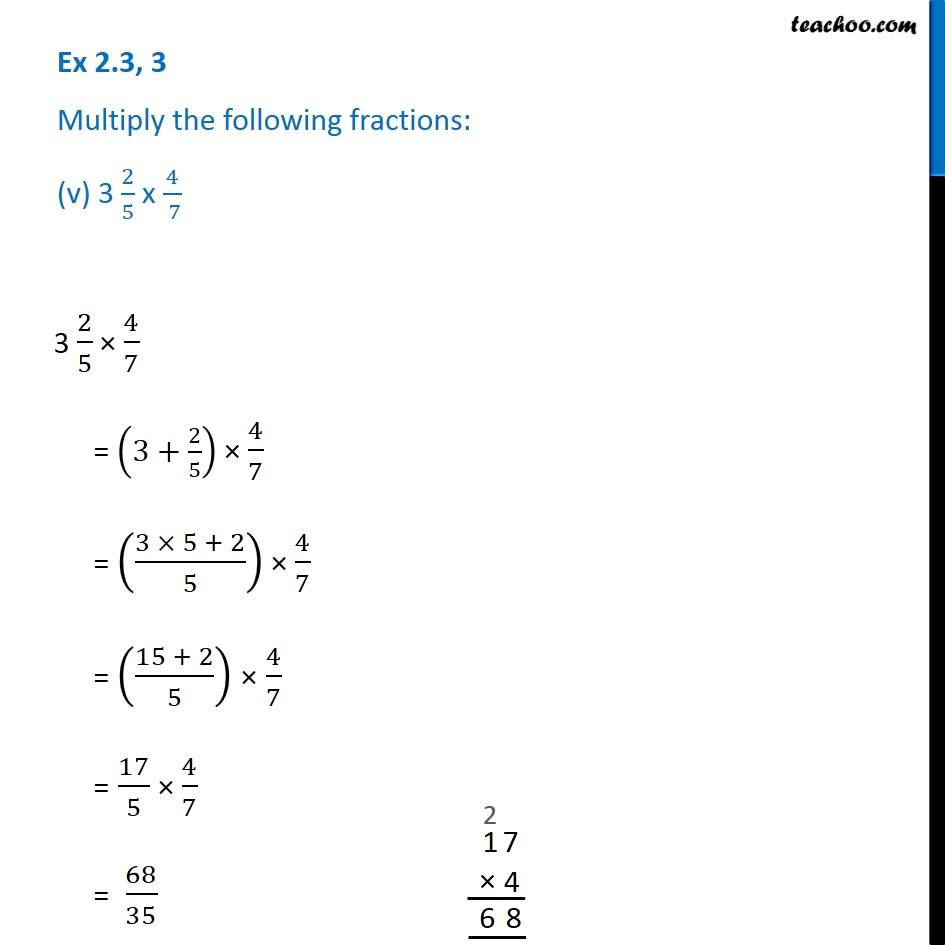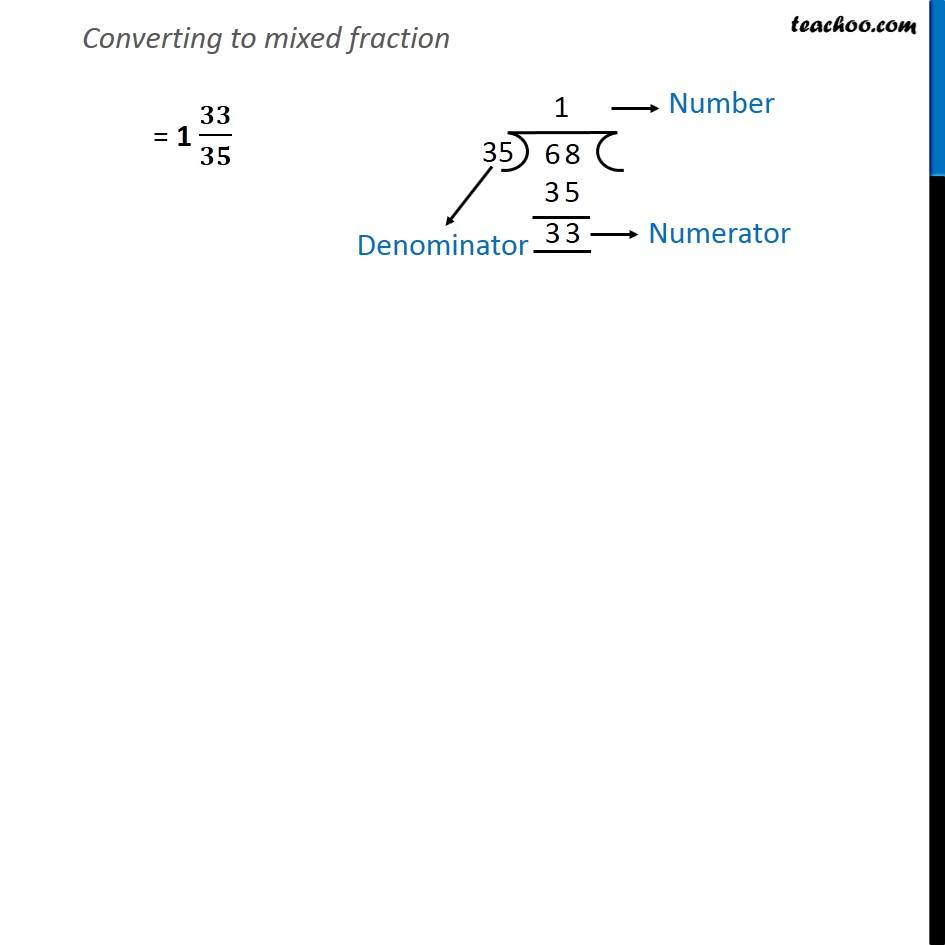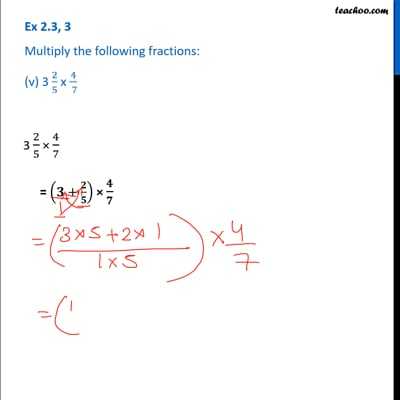Ex 2.3

Chapter 2 Class 7 Fractions and Decimals
Serial order wiseThis video is only available for Teachoo black users

Get live Maths 1-on-1 Classs - Class 6 to 12

### Transcript

Ex 2.3, 3 Multiply the following fractions: (v) 3 2/5 x 4/( 7) 3 2/5 × 4/7 = (3+2/5) × 4/7 = ((3 × 5 + 2)/5) × 4/7 = ((15 + 2)/5) × 4/7 = 17/5 × 4/7 = 68/35 Converting to mixed fraction = 1 𝟑𝟑/𝟑𝟓